### Home > MC1 > Chapter 4 > Lesson 4.3.2 > Problem4-99

4-99.
1. . The following number lines are missing some numbers. Use the information provided to complete the number lines. Homework Help ✎

1.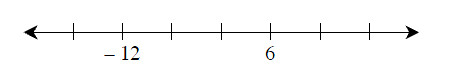2.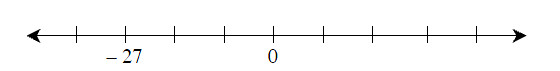3.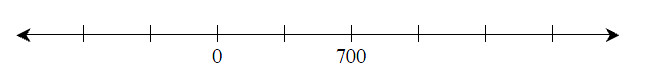4.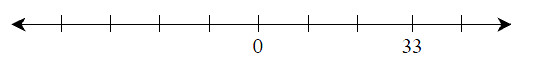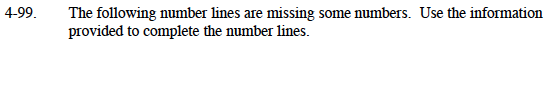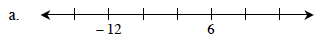Start by finding the difference in one space.

To do this, find the difference between the two given numbers and divide that by the number of spaces between them.

The difference between −12 and 6 is 18.

$\frac{18}{3}=6.$

The difference in one space is 6.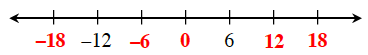The number line has been filled in above for you.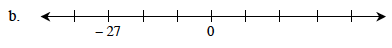Follow the steps in part (a).

The difference between 0 and −27 is 27.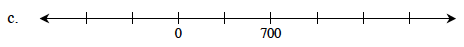Follow the steps in part (a).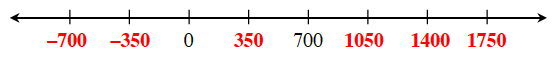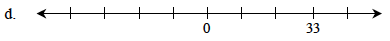Follow the steps in part (a).